This follows chpt2 of "Programming Collective Intelligence", by T. Segaran: ("Ask your friends, weight according to similarity...")

In :
from scipy import stats
#r,p=stats.pearsonr(xdata,ydata)
#slope, intercept, r_value, p_value, std_err = stats.linregress(xdata,ydata)
from collections import defaultdict

In :
# A dictionary of movie reviewers and their ratings of a small set of movies
reviews={
'Lisa Rose':
{'Lady in the Water': 2.5, 'Snakes on a Plane': 3.5, 'Just My Luck': 3.0,
'Superman Returns': 3.5, 'You, Me and Dupree': 2.5, 'The Night Listener': 3.0},
'Gene Seymour':
{'Lady in the Water': 3.0, 'Snakes on a Plane': 3.5, 'Just My Luck': 1.5,
'Superman Returns': 5.0, 'The Night Listener': 3.0, 'You, Me and Dupree': 3.5},
'Michael Phillips':
{'Lady in the Water': 2.5, 'Snakes on a Plane': 3.0,
'Superman Returns': 3.5, 'The Night Listener': 4.0},
'Claudia Puig':
{'Snakes on a Plane': 3.5, 'Just My Luck': 3.0, 'The Night Listener': 4.5,
'Superman Returns': 4.0, 'You, Me and Dupree': 2.5},
'Mick LaSalle':
{'Lady in the Water': 3.0, 'Snakes on a Plane': 4.0, 'Just My Luck': 2.0,
'Superman Returns': 3.0, 'The Night Listener': 3.0, 'You, Me and Dupree': 2.0},
'Jack Matthews':
{'Lady in the Water': 3.0, 'Snakes on a Plane': 4.0,
'The Night Listener': 3.0, 'Superman Returns': 5.0, 'You, Me and Dupree': 3.5},
'Toby':
{'Snakes on a Plane':4.5,'You, Me and Dupree':1.0,'Superman Returns':4.0}
}

In :
len(reviews), map(len,reviews.values())

Out:
(7, [5, 6, 5, 6, 3, 6, 4])
In :
#what has Toby reviewed:
reviews['Toby']

Out:
{'Snakes on a Plane': 4.5, 'Superman Returns': 4.0, 'You, Me and Dupree': 1.0}
In :
# plot the critics who reviewed these two:
dupree='You, Me and Dupree'
snakes='Snakes on a Plane'

crit2 = [critic for critic in reviews if dupree in reviews[critic] and snakes in reviews[critic]]
du_ratings=[reviews[c][dupree] for c in crit2]
sn_ratings=[reviews[c][snakes] for c in crit2]

figure(figsize=(4.5,4.5))
xlim(.9,5.1),ylim(.9,5.1)
xlabel(dupree), ylabel(snakes)

plot(du_ratings,sn_ratings,'bo')

for crit,x,y in zip(crit2,du_ratings,sn_ratings):
print '{}: ({},{})'.format(crit,x,y)
#move Puig down to avoid collision
text(x+.05,y+(.01 if 'Puig' not in crit else -.2),crit.split()[-1])

Jack Matthews: (3.5,4.0)
Mick LaSalle: (2.0,4.0)
Claudia Puig: (2.5,3.5)
Lisa Rose: (2.5,3.5)
Toby: (1.0,4.5)
Gene Seymour: (3.5,3.5)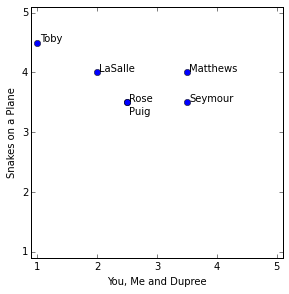Who's close to whom in the above fig? Based on just these two movies, we'd conclude that Rose and Puig are quite close (exact agreement), whereas Toby is quite different from Seymour, differing by 2.5 on one movie and 1 on the other. The distance between them on the plot above is $\sqrt{(3.5-1)^2+(4.5-3.5)^2}\approx2.69$. For critics who have co-rated multiple movies, this notion of distance generalizes to taking the square root of the sum of the squares of all of those co-rated movies, $d^2_{ij}=\sum_m\bigl(r_m(i) - r_m(j)\bigr)^2$. (This corresponds to the Euclidean distance between $r_m(i)$ and $r_m(j)$, considered as vectors in an M-dimensional space, $d^2_{ij}=\bigl(\vec r(i)-\vec r(j)\bigr)^2$.)

It is convenient to employ a measure of similarity rather than of distance (the two are inversely related), defined as $s_{ij}={1\over 1+d_{ij}^2}$. The similarity $s_{ij}$ goes from 0 similarity when the distance is very large, up to similarity 1 when the distance goes to zero. Here sim_distance() returns this similarity score for two reviewers, based only on the items they've both rated:

In :
def sim_distance(prefs,person1,person2):
# Get the list of shared_items
shared_items=[item for item in prefs[person1] if item in prefs[person2]]

# if they have no ratings in common, return 0
if len(shared_items)==0: return 0

v1=array([prefs[person1][item] for item in shared_items])
v2=array([prefs[person2][item] for item in shared_items])

# use numpy euclidean distance (sqrt(sum of squares))
dist=norm(v1-v2)

#transform to similarity ranging from 0 to 1
#truncate to three after decimal point
return 1/(1+dist**2)

In :
#'Lisa Rose' happened to rate all movies
all_movies = sorted(reviews['Lisa Rose'],key=reviews['Lisa Rose'].get)

def ratings(critic): return array([reviews[critic][m] for m in all_movies])

for crit in ('Lisa Rose','Gene Seymour','Mick LaSalle'):
print crit,ratings(crit)
plot(ratings(crit),'o-',label=crit)

ylim(0,6),xlim(-.5,5.5)
legend(loc='upper left');

Lisa Rose [ 2.5  2.5  3.   3.   3.5  3.5]
Gene Seymour [ 3.   3.5  1.5  3.   3.5  5. ]
Mick LaSalle [ 3.  2.  2.  3.  4.  3.]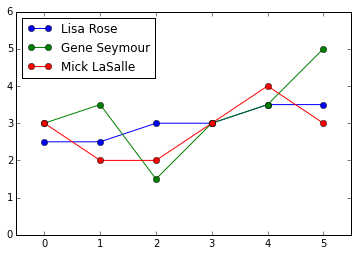In :
#for example, the green and blue
round(sim_distance(reviews,'Lisa Rose','Gene Seymour'),3)

Out:
0.148

Consider a different distance measure: Pearson correlation coefficient (+1 if correlated, -1 if anticorrelated, and values in between for noisy correlation)

Recall from power_law.ipynb that stats.linregress() also returned an r_value, known as the "Pearson correlation coefficient", defined as

$$\qquad {E[(X-E[X])(Y-E[Y])]\over \sigma_X\sigma_Y}$$

Note that Pearson corrects for "grade inflation", unlike euclidean distance: one can be systematically higher than the other, offset won't matter. The numerator is the "covariance" of X and Y, equal to a times the variance of X when Y=aX+b. The denominator is equal to the absolute value |a| times the variance when Y=aX+b, so the value of the Pearson correlation coefficient is $\pm1$ for an exact linear relation (where the sign depends on the sign of the slope a). For noisier data the value is between -1 and 1, with 0 corresponding to no correlation.

In :
from IPython.display import Image
Image('Pearson.png',width=600)

Out: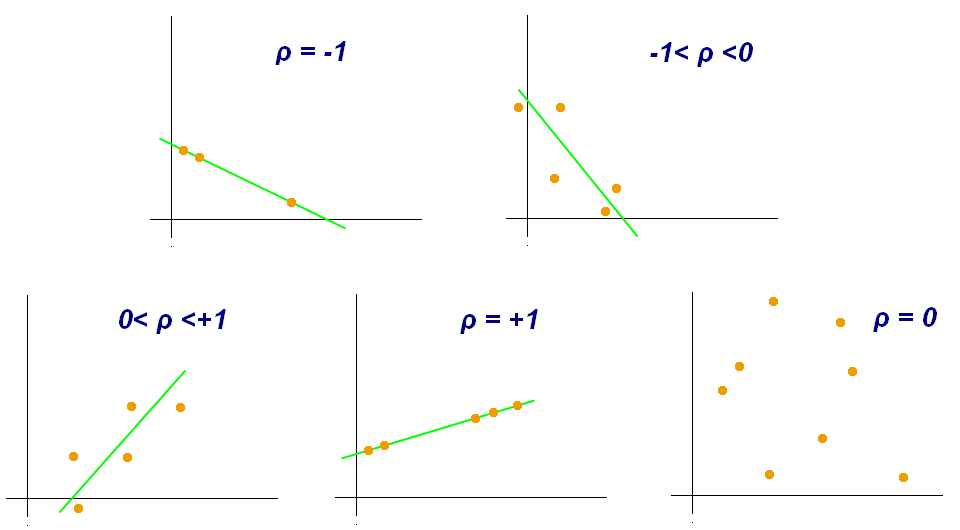In :
#three critics who rated all 6
crit6 = [crit for crit in reviews if len(reviews[crit])==6]
#three pairs from above set
pairs = [(crit6[i],crit6[j]) for i in range(len(crit6)) for j in range(i)]

In :
#compare the similarity based on distance with Pearson for these three pairs:

figure(figsize=(10.5,6.6))
for j,(c1,c2) in enumerate(pairs):
subplot(2,3,j+1)
scatter(ratings(c1),ratings(c2))
for (x,y) in zip(ratings(c1),ratings(c2)): plot((x,x),(x,y),'r-',marker='_',ms=10)
grid('on')
xticks(range(1,6)),yticks(range(1,6))
axis((.9,5.1,.9,5.1))
xlabel(c1),ylabel(c2)
plot((1,5),(1,5),'b--')
d=norm(ratings(c1)-ratings(c2))
text(1,4.25,'sim_distance={:.2f}\n(distance={:.2f})'.\
format(sim_distance(reviews,c1,c2),d),fontsize=12,color='b')

subplot(2,3,j+4)
scatter(ratings(c1),ratings(c2))
xticks(range(1,6)),yticks(range(1,6))
axis((.9,5.1,.9,5.1))
xlabel(c1),ylabel(c2)
r,p=stats.pearsonr(ratings(c1),ratings(c2))
text(1,4.25,'Pearson r={:.2f}\n(p={:.2f})'.format(r,p),fontsize=12,color='b')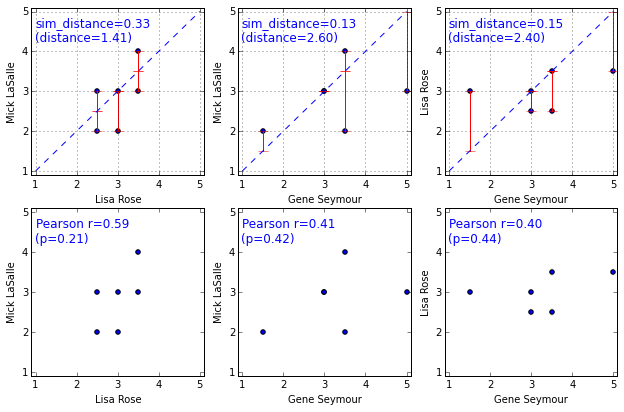In :
#define function to look at the Pearson r for a few critic pairs

def show_pearson(prefs,crit1,crit2):
shared_items=[item for item in prefs[crit1] if item in prefs[crit2]]

figure(figsize=(5,5))
xlim(.8,5.2),ylim(.8,5.2)
xdata = [prefs[crit1][item] for item in shared_items]
ydata = [prefs[crit2][item] for item in shared_items]

slope, intercept, r_value, p_value, std_err = stats.linregress(xdata,ydata)
xlabel(crit1),ylabel(crit2)

plot(xdata,ydata,'o')
plot(slope*arange(6)+intercept,'--')

voffset={(x,y):.01 for x,y in zip(xdata,ydata)}
for item in shared_items:
x,y=prefs[crit1][item],prefs[crit2][item]
text(x+.05, y+voffset[(x,y)], item)
voffset[(x,y)]-=.15
text(1.25,4.5,'Pearson r = {:.2f}'.format(r_value),fontsize=14,color='b')

In :
#two fake critics roughly correlated
show_pearson(fcritics,'critic1','critic2')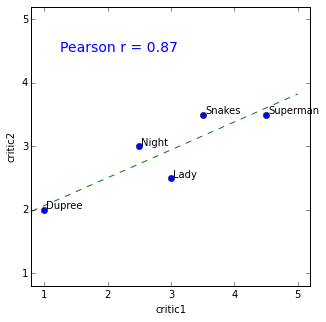In :
#two from original set not quite as well correlated
show_pearson(reviews,'Mick LaSalle','Gene Seymour')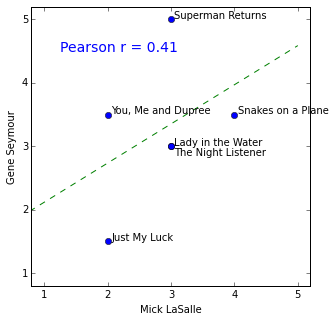In :
#now define similarity measure, analogous to sim_distance
def sim_pearson(prefs,crit1,crit2):
shared_items=[item for item in prefs[crit1] if item in prefs[crit2]]
#  shared_items=list(set(prefs[person1]) & set(prefs[person2]))  #equivalent
if len(shared_items)==0: return 0
xdata = [prefs[crit1][item] for item in shared_items]
ydata = [prefs[crit2][item] for item in shared_items]
r,p=stats.pearsonr(xdata,ydata)
if isnan(r): return 0
return r


Now rank the critics, find other critics similar to given person ranked according to similarity measure:

In :
# Returns the best matches for person from the prefs dictionary.
# Number of results and similarity function are optional params.

def topMatches(prefs, person, n=5, similarity=sim_pearson):
scores=[(other, round(similarity(prefs,person,other),3))
for other in prefs if other != person]
return sorted(scores,key=lambda x:x,reverse=True)[:n]

In :
topMatches(reviews,'Toby',6)

Out:
[('Lisa Rose', 0.991),
('Mick LaSalle', 0.924),
('Claudia Puig', 0.893),
('Jack Matthews', 0.663),
('Gene Seymour', 0.381),
('Michael Phillips', -1.0)]
In :
# see how topmatches function works using other similarity measure
topMatches(reviews,'Toby', n=3, similarity=sim_distance)

Out:
[('Mick LaSalle', 0.308), ('Michael Phillips', 0.286), ('Claudia Puig', 0.235)]

But here what we really want is to make a recommendation. For this, we could use just the most similar person, and select any movies that person liked that you haven't seen. But that person might not have seen the most relevant movie, or might be an outlier on that particular movie (i.e., liked it but most people with tastes similar to yours didn't like it, or vice versa).

So instead, get recommendations by using a weighted average of every other person's ratings, weighted according to that person's similarity to you. Suppose person $i$ has similarity $s_i$ to you, and gives rating $r_i(m)$ to movie $m$ that you haven't seen, then that person will contribute $s_i\cdot r_i(m)$ to your likely preference for movie $m$, so that others' ratings are weighted in proportion to their similarity to you. Then we sum on $i$ over all those who have rated movie $m$, and divide by the same sum over the $s_i$ to give the expected value of your rating of movie $m$:

$$\qquad\qquad E[r_m]={\sum_{i\ |\ i\ {\rm rated\ }m} s_i\cdot r_m(i)\over \sum_{i\ |\ i\ {\rm rated\ }m} s_i}$$

This equation is implemented in the function below, and gives the expected rating of all movies one hasn't seen:

In :
def getRecommendations(prefs,person,similarity=sim_pearson):
totals={}
simSums={}
for other in prefs:
# don't compare me to myself
if other==person: continue
sim=similarity(prefs,person,other)

# ignore scores of zero or lower
if sim<=0: continue
for item in prefs[other]:

# only score movies I haven't seen yet
if item not in prefs[person] or prefs[person][item]==0:
# Similarity * Score
if item not in totals:
totals[item]=0
simSums[item]=0
totals[item] += prefs[other][item]*sim
# Sum of similarities
simSums[item] += sim

# Create the normalized list
rankings=[(item,round(totals[item]/simSums[item],3)) for item in totals]

# Return the sorted list
return sorted(rankings,key=lambda x:x,reverse=True)

In :
getRecommendations(reviews,'Toby')
#also gives likely rating

Out:
[('The Night Listener', 3.348),
('Just My Luck', 2.531)]
In :
#or use other distance measure
getRecommendations(reviews,'Toby',similarity=sim_distance)

Out:
[('The Night Listener', 3.5),
('Just My Luck', 2.462)]

Now suppose you want matching products, e.g., Amazon "customers have also bought". Now instead of similarity between people based on the ratings given to some object, averaged over objects both have rated, now we consider similarity between objects that are rated, based on whether different reviewers tend to rate them similarly. This is equivalent to reversing the roles of reviewers and objects, so that it is now the objects that are rating the reviewers:

In :
#first reverse role of items and objects
def transformPrefs(prefs):
result=defaultdict(dict)
for person in prefs:
for item in prefs[person]:
# Flip item and person
result[item][person]=prefs[person][item]
return result


Try this for the movie ratings above, to get similarities between movies:

In :
movies=transformPrefs(reviews)
movies

Out:
{'Just My Luck': {'Claudia Puig': 3.0,
'Gene Seymour': 1.5,
'Lisa Rose': 3.0,
'Mick LaSalle': 2.0},
'Lady in the Water': {'Gene Seymour': 3.0,
'Jack Matthews': 3.0,
'Lisa Rose': 2.5,
'Michael Phillips': 2.5,
'Mick LaSalle': 3.0},
'Snakes on a Plane': {'Claudia Puig': 3.5,
'Gene Seymour': 3.5,
'Jack Matthews': 4.0,
'Lisa Rose': 3.5,
'Michael Phillips': 3.0,
'Mick LaSalle': 4.0,
'Toby': 4.5},
'Superman Returns': {'Claudia Puig': 4.0,
'Gene Seymour': 5.0,
'Jack Matthews': 5.0,
'Lisa Rose': 3.5,
'Michael Phillips': 3.5,
'Mick LaSalle': 3.0,
'Toby': 4.0},
'The Night Listener': {'Claudia Puig': 4.5,
'Gene Seymour': 3.0,
'Jack Matthews': 3.0,
'Lisa Rose': 3.0,
'Michael Phillips': 4.0,
'Mick LaSalle': 3.0},
'You, Me and Dupree': {'Claudia Puig': 2.5,
'Gene Seymour': 3.5,
'Jack Matthews': 3.5,
'Lisa Rose': 2.5,
'Mick LaSalle': 2.0,
'Toby': 1.0}}
In :
#now topmatches gives similar movies rather than similar reviewers
topMatches(movies,'Superman Returns')

Out:
[('You, Me and Dupree', 0.658),
('Snakes on a Plane', 0.112),
('The Night Listener', -0.18),
('Just My Luck', -0.423)]
In :
#note negative scores, reviewers who like one dislike the other
show_pearson(movies,'Just My Luck','Superman Returns')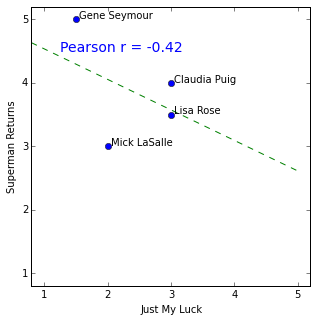Finally, the analog of the recommended movie for a given person, after reversing the roles of people and movies, is the recommended person for a movie, i.e., the one most likely to like it:

In :
getRecommendations(movies,'Just My Luck')
#find critics for movie ... invite to premiere?

Out:
[('Michael Phillips', 4.0), ('Jack Matthews', 3.0)]

It's easy to apply these to de.licio.us data (details in the chpt2 of text mentioned at top). python code to interact with delicious api is here: http://code.google.com/p/pydelicious/source

Also works with real movie datasets from movielens, http://www.grouplens.org/datasets/movielens/

In [ ]: